# GMAT Math : DSQ: Calculating if two acute / obtuse triangles are similar

## Example Questions

### Example Question #4 : Triangles

Which of two triangles has greater area,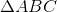or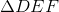?

Statement 1: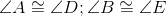Statement 2: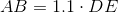Statement 2 ALONE is sufficient to answer the question, but Statement 1 ALONE is NOT sufficient to answer the question.

Statement 1 ALONE is sufficient to answer the question, but Statement 2 ALONE is NOT sufficient to answer the question.

BOTH statements TOGETHER are sufficient to answer the question, but NEITHER statement ALONE is sufficient to answer the question.

EITHER statement ALONE is sufficient to answer the question.

BOTH statements TOGETHER are insufficient to answer the question.

BOTH statements TOGETHER are sufficient to answer the question, but NEITHER statement ALONE is sufficient to answer the question.

Explanation:

Statement 1 alone proves that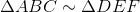by the Angle-Angle Postulate, but does not prove anything about the sides, which would be needed to answer this question. Statement 2 alone only gives a relationship between one side of each triangle; without any further information, this is insufficient.

The two statements together, however, present sufficient evidence. Statement 1 proves that the triangles are similar. Statement 2 gives the ratio of one side into the corresponding side in, so, as the triangles are similar, this ratio is shared by all three pairs of corresponding sides. Since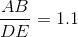,

1.1 is this common ratio, and the ratio of the area oftois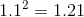, makingthe larger triangle.

### Example Question #5 : Triangles

True or false:Statement 1: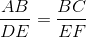Statement 2: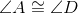and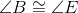Statement 1 ALONE is sufficient to answer the question, but Statement 2 ALONE is NOT sufficient to answer the question.

Statement 2 ALONE is sufficient to answer the question, but Statement 1 ALONE is NOT sufficient to answer the question.

BOTH statements TOGETHER are sufficient to answer the question, but NEITHER statement ALONE is sufficient to answer the question.

BOTH statements TOGETHER are insufficient to answer the question.

EITHER statement ALONE is sufficient to answer the question.

Statement 2 ALONE is sufficient to answer the question, but Statement 1 ALONE is NOT sufficient to answer the question.

Explanation:

Statement 1 alone gives a proportion between two pairs of corresponding sides of the triangles. This is not enough to prove the triangles similar without the third side proportionality (SSS Simiilarity statement) or the congruence of the included angles (SAS Similarity statement).

Statement 2 gives two congruencies between corresponding angles, which by the Angle-Angle statement is enough to prove the triangles similar.

Tired of practice problems?

Try live online GMAT Math prep today.